Maths-
General
Easy

Question

# What sum of money lent out at 5% for 3 years will produce the same interest as Rs. 900 lent out at 4% for 5 years.Hint:

## The correct answer is: 1200 Rupees

### Complete step by step solution:We calculate simple interest by the formula,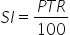…(i)where P is Principal amount, T is number of years and R is rate of interestCase ⅠLet the sum of money = PHere, we have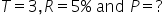On substituting the values in (i), we get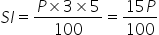…(ii)Case ⅡHere, we have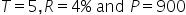On substituting the values in (i), we get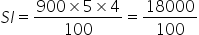…(iii)It is given that the interest produced in both the cases is the same.So, Equate (ii) and (iii)On equating, we get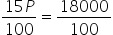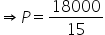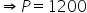rupees.Hence the sum of money P = 1200 Rupees#### With Turito Foundation.#### Get an Expert Advice From Turito.